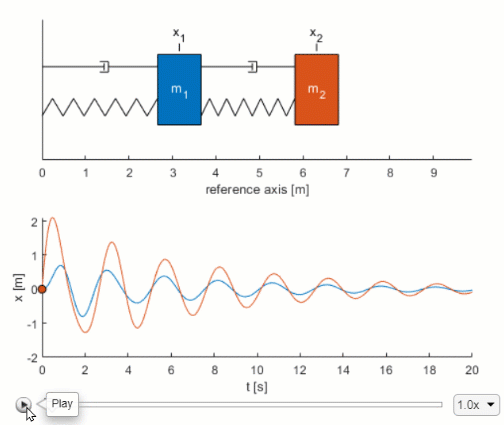## Mass-Spring-Damper Systems

version 1.0.0 (7.05 MB) by
Interactive courseware module that addresses the fundamentals of mass-spring-damper systems taught in mechanical engineering courses.

Updated 21 Jun 2021

From GitHub

# Mass-Spring-Damper SystemsCurriculum Module
Created with R2020b. Compatible with R2020b and later releases.## Description

This curriculum module contains interactive live scripts and Simulink® models that explore mass-spring-damper systems. Students learn to create and work with mass-spring-damper models in guided activities. These techniques are motivated by two applications: tuning the damping of a vehicle’s suspension and analyzing a building’s response to an earthquake. Throughout the module, students apply Simulink models to study the dynamics of the physical systems. In the final lesson, students identify the resonant frequencies of a mass-spring-damper building model by computing the power spectrum of a displacement signal. These lessons can be used as part of a lecture, as activities in an instructional setting, or as interactive assignments to be completed outside of class.

Learning Goals

• Construct free body diagrams and derive the equations of motion for mass-spring-damper systems
• Relate the mass, spring, and damper to their corresponding components in a physical system
• Create models that solve ordinary differential equations in Simulink
• Use the Symbolic Math Toolbox to help create Simulink models
• Complete Simulink mass-spring-damper models with 1, 2, and n degrees of freedom
• Relate parameter values to the dynamics of mass-spring-damper systems
• Tune the damping of a vehicle suspension model to meet requirements
• Identify the resonant modes of a mass-spring-damper building model

The instructions inside the live scripts will guide you through the exercises and activities. Get started with each live script by running it one section at a time. To stop running the script or a section midway (for example, when an animation is in progress), use the Stop button in the RUN section of the Live Editor tab in the MATLAB Toolstrip.

## Suggested Prework

MATLAB Onramp – a free two-hour introductory tutorial that teaches the essentials of MATLAB.
Simulink Onramp – a free three-hour introductory tutorial that teaches the essentials of Simulink.

## Details

`massSpringDamper.mlx, massSpringDamperSoln.mlx`
An interactive lesson that teaches how to model a single mass-spring-damper in Simulink. Students apply their knowledge to tune the damping of a vehicle’s suspension system.

`doubleMassSpringDamper.mlx, doubleMassSpringDamperSoln.mlx`
An interactive lesson that teaches how to model a double mass-spring-damper in Simulink. Students apply their knowledge to identify the resonant frequencies present in a two-story building model.

`multipleMassSpringDamper.mlx, multipleMassSpringDamperSoln.mlx`
Students practice more advanced skills in this lesson, such as vectorizing a Simulink model and using the Spectrum Analyzer block to compute the power spectrum.

`models/`
Simulink models used during the guided activities. Starter models and their completed solutions are included.

## Products

The license for this module is available in the LICENSE.TXT file in this GitHub repository.

## Educator Resources

Have any questions or feedback? Contact the MathWorks online teaching team.

### Cite As

Brian Hong (2021). Mass-Spring-Damper Systems (https://github.com/MathWorks-Teaching-Resources/Mass-Spring-Damper-Systems/releases/tag/v1.0.0), GitHub. Retrieved .

##### MATLAB Release Compatibility
Created with R2020b
Compatible with R2020b and later releases
##### Platform Compatibility
Windows macOS Linux